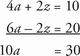# SAT Math Multiple Choice Question 225: Answer and Explanation

### Test Information

Question: 225

15. 4a + 2z = 10
-3a + z = -10

Based on the system of equations above, what is the value of 10a ?

• A. -10
• B. -1
• C. 3
• D. 30